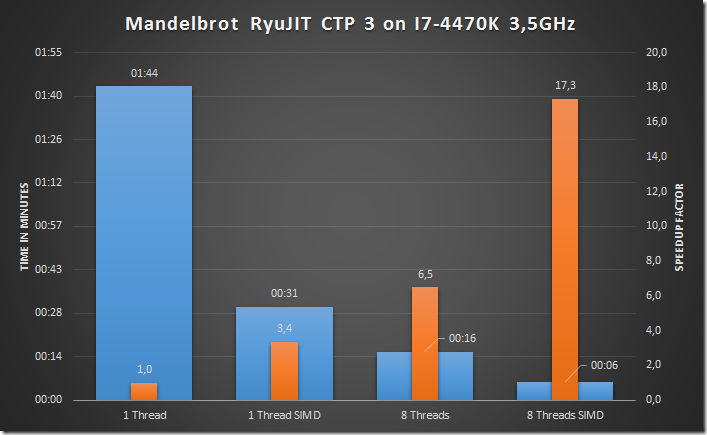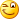# Alois Kraus

blog

Home  |   Contact  |   Syndication|   Login### Programming

Finally after long years of stagnation the JIT compiler got significant attention to support SIMD instructions. As an experienced .NET Developer your might think SIMD? What? Never heard of! Don´t worry: You will hear the same from most experienced C/C++ developers. SIMD is more a geek thing for performance addicts which stands for Single Instruction Multiple Data.

SIMD support means that the compiler (Intel, MSVC++ and now .NET JIT) can emit assembly instructions that take advantage of CPU features which can execute one operation (e.g. add) on multiple inputs at once. Why should I care? My Core I7 has 4 physical cores (8 with hyper threading). Now lets add to the mandelbrot sample SIMD support.Multithreading stays important but when you can get with a single core a speedup of 3,4 simply by employing SIMD. That is something you cannot ignore. If your server today needs 40 cores and you can cut the number of cores down by a factor 3,4. You can spend the saved money on other things than hardware (e.g. paying developers to program SIMD enabled code). This is all nice and well but how fast can it get? When I look at the generated assembler code

d:\Source\SIMDSample\C#\Mandelbrot\VectorFloatStrict.cs @ 162:
00007ffd`e99d2139 4183461004      add     dword ptr [r14+10h],4
00007ffd`e99d213e 410f28c6        movaps  xmm0,xmm14
00007ffd`e99d2142 410f28c8        movaps  xmm1,xmm8
00007ffd`e99d2146 660fefd2        pxor    xmm2,xmm2
00007ffd`e99d214a 0f28d0          movaps  xmm2,xmm0
00007ffd`e99d214d 0fc2d102        cmpleps xmm2,xmm1
00007ffd`e99d2151 660f76c0        pcmpeqd xmm0,xmm0
00007ffd`e99d2155 0f28ca          movaps  xmm1,xmm2
00007ffd`e99d2158 660f76c8        pcmpeqd xmm1,xmm0
00007ffd`e99d215c 660f70d94e      pshufd  xmm3,xmm1,4Eh

I see these xmm registers. These are SSE 128 bit wide registers which are the foundations for the current SIMD support of the JIT compiler. But this is still not the end. My Haswell CPU supports AVX2 which can cope with 256 bit wide registers which should give me a speedup of 8. AVX-512 will arrive in some time giving us 512 bit wide registers. We can achieve then speedup a single thread up to factor of 16! In theory we should be able to get this graph with an AVX-512 enabled CPU and a perfect .NET JIT compilerFor some problem domains it can happen that SIMD support may be more important than using more threads. You can cope with heavy load on a single core without the need to use many threads. That can help servers which are shared by many users a lot. Here are the facts how to get started with SIMD in .NET:

• .NET uses SIMD automatically for my application?

No. You need to use a specialized SIMD library which offers SIMD data types which can be translated by the JIT compiler to SIMD instructions on CPUs supporting it.

• Can I use the normal .NET Framework?

• Does it work on Debug Builds?

No. SIMD instructions are only used for Release builds with RyuJIT enabled (COMPLUS_AltJit=*) and SIMD instructions enabled (COMPLUS_FeatureSIMD=1) for this CTP on Windows 8 and above for x64 applications only.

• How looks SIMD vs non SIMD code?

Here is the "normal" Modelbrot core method

C#\Mandelbrot\ScalarFloat.cs

```        // Render the fractal with no data type abstraction on a single thread with scalar floats
public void RenderSingleThreadedNoADT(float xmin, float xmax, float ymin, float ymax, float step)
{
int yp = 0;
for (float y = ymin; y < ymax && !Abort; y += step, yp++)
{
int xp = 0;
for (float x = xmin; x < xmax; x += step, xp++)
{
float accumx = x;
float accumy = y;
int iters = 0;
float sqabs = 0f;
do
{
float naccumx = accumx * accumx - accumy * accumy;
float naccumy = 2.0f * accumx * accumy;
accumx = naccumx + x;
accumy = naccumy + y;
iters++;
sqabs = accumx * accumx + accumy * accumy;
} while (sqabs < limit && iters < max_iters);
DrawPixel(xp, yp, iters);
}
}
}```

And here is the SIMD version. Not very different but the performance differs a lot. If you use double types the benefit will be smaller (factor 2) because double uses 8 bytes of memory whereas single precision (float) values use up only 4 bytes. You might ask what is this version with the strange name NoADT? It means No Abstract Data Types. The other version uses its own Complex data type which is a lot slower. This should be improved in future CTPs either by getting our BCL enabled Complex data type or by optimizing custom data types which contain SIMD types in general.

C#\Mandelbrot\VectorFloat.cs

```        // Render the fractal on a single thread using raw Vector<float> data types
public void RenderSingleThreadedNoADT(float xmin, float xmax, float ymin, float ymax, float step)
{
Vector<float> vmax_iters = new Vector<float>(max_iters);
Vector<float> vlimit = new Vector<float>(limit);
Vector<float> vstep = new Vector<float>(step);
Vector<float> vxmax = new Vector<float>(xmax);
Vector<float> vinc = new Vector<float>((float)Vector<float>.Length * step);
Vector<float> vxmin = VectorHelper.Create(i => xmin + step * i);

float y = ymin;
int yp = 0;
for (Vector<float> vy = new Vector<float>(ymin); y <= ymax && !Abort; vy += vstep, y += step, yp++)
{
int xp = 0;
for (Vector<float> vx = vxmin; Vector.LessThanOrEqualAll(vx, vxmax); vx += vinc, xp += Vector<int>.Length)
{
Vector<float> accumx = vx;
Vector<float> accumy = vy;

Vector<float> viters = Vector<float>.Zero;
Vector<float> increment = Vector<float>.One;
do
{
Vector<float> naccumx = accumx * accumx - accumy * accumy;
Vector<float> naccumy = accumx * accumy + accumx * accumy;
accumx = naccumx + vx;
accumy = naccumy + vy;
viters += increment;
Vector<float> sqabs = accumx * accumx + accumy * accumy;
Vector<float> vCond = Vector.LessThanOrEqual<float>(sqabs, vlimit) &
Vector.LessThanOrEqual<float>(viters, vmax_iters);
increment = increment & vCond;
} while (increment != Vector<float>.Zero);

viters.ForEach((iter, elemNum) => DrawPixel(xp + elemNum, yp, (int)iter));
}
}
}```

This is a long awaited step into the right direction of the JIT compiler. Finally you can write performance sensitive code without much hassle in .NET as well. Still the JIT compiler could become smarter for many common code constructs to emit more efficient code.

posted on Friday, April 4, 2014 11:46 AM